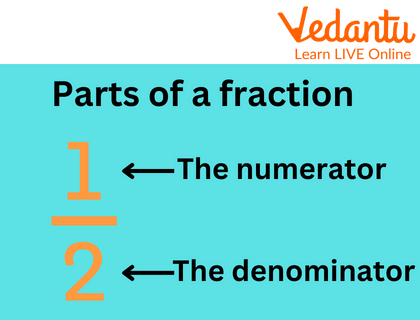Courses
Courses for Kids
Free study material
Free LIVE classes
More

# Fraction For KidsLIVE
Join Vedantu’s FREE Mastercalss

## What is a Fraction?

A fraction is a whole or part of a set of objects. A fraction consists of two parts separated by a line. The number present at the top of the line is the numerator. It tells you how many equal parts of a whole or set are. The number present below the line is the denominator. Displays the total number of equal parts divided by the whole or the total number of equal objects in a collection.Example Of Fraction

## Parts of a Fraction

### Every fraction has a numerator and a denominator divided by a horizontal bar defined as the fractional bar.

• The denominator represents the number of components into which the whole has been subdivided. It is positioned below the fractional bar at the lowest section of the fraction.

• The numerator specifies how many fractional parts are depicted or selected. It is positioned above the fractional bar at the upper section of the fraction.

For example, $\dfrac{5}{7}$ in this fraction, the numerator 5 is, and the denominator is 7.Parts of a Fraction

## Types of Fraction With Example

The different types of fractions depend on the numerator and denominator.

• Proper fraction - Proper fractions are the ones whose numerator is less than the denominator is called a proper fraction. For example, $\dfrac{2}{5},\dfrac{{15}}{{16}}$.

• Improper fraction - Fractions whose numerator is greater than or equal to the denominator are called improper fractions. For example,  $\dfrac{7}{3},\dfrac{{45}}{{13}}$ are improper fractions.

• Mixed fractions - A mixed fraction contains a whole number and a proper fraction. For example, $8\dfrac{{13}}{{20}}$. .

• Like fractions - If two or more fractions have the same denominator, they are said to be like fractions. For example, $\dfrac{3}{{11}},\dfrac{5}{{11}},\dfrac{7}{{11}}$ all have the same denominator 11.

• Unlike fractions - When two or more fractions have different denominators, they are said to be unlike fractions. For example, $\dfrac{5}{9},\dfrac{7}{{11}},\dfrac{9}{{13}}$  have different denominators. If fractions are unlike when adding or subtracting fractions, they are converted to like fractions.

• Equivalent fraction - Equal fractions are fractions whose numerator and denominator are different but have the same value when simplified or reduced. So, fractions of equal value are called equivalent fractions.  For example, $\dfrac{4}{8},\dfrac{{11}}{{22}},\dfrac{{12}}{{24}}$ are equivalent fractions because they all reduce to $\dfrac{1}{2}$.

• Unit fraction - A fraction is a fraction whose numerator is 1, and the denominator is a positive integer. For example,  $\dfrac{1}{2},\dfrac{1}{{47}}$ etc. are called unit fractions.

## Conclusion

Fractions are the particular part of a whole number. The part above the bar is the numerator, and the part below the bar is the denominator. Different types of fractions are based on their numerator and denominator. We must change the fractions into like fractions to apply any arithmetic operation like addition or subtraction.

## Solved Examples

1. The fractions $\dfrac{4}{{12}},\dfrac{8}{{24}},\dfrac{{11}}{{33}}$ are

a. like fractions

b. unlike fractions

c. equivalent fractions

d. unit fractions

Ans: Equivalent fraction

Explanation: The fractions are equivalent as when they have simplified, the result we get is $\dfrac{1}{3}$, making them an equivalent fraction.

2. The reciprocal of $\dfrac{{10}}{{78}}$ will be

a. $\dfrac{1}{{78}}$

b. $\dfrac{{78}}{{10}}$

c. $\dfrac{{100}}{{78}}$

d. $\dfrac{{10}}{{780}}$

Ans: $\dfrac{{78}}{{10}}$

Explanation: The reciprocal of the number can be done when we reverse the place of the numerator with the denominator and the denominator with the numerator.

3. The fractions $\dfrac{1}{{56}},\dfrac{1}{2},\dfrac{1}{8}$ are

a. like fractions

b. unlike fractions

c. equivalent fractions

d. unit fractions

Ans: Unit fractions

Explanation: The fractions with 1 in their numerator are known as unit fractions.

Last updated date: 27th Sep 2023
Total views: 77.4k
Views today: 1.77k

## FAQs on Fraction For Kids

1. What is the condition for the fraction to be a proper fraction?

The condition for any fraction to be a proper fraction is that it should have a denominator greater than the numerator itself.

2. Can a fraction have 0 in its numerator?

No, a fraction cannot have 0 in its numerator. The value of any fraction having 0 in its numerator always equals 0.

3. Is every fraction less than one?

No, not all of the fractions are smaller than one. Proper fractions are higher than 0 but less than 1 because the denominator is larger than the numerator. Improper fractions are always one or more than one because the numerator exceeds or equals the denominator.

4. How Do You Multiply Fractions?

To multiply any consecutive fractions, multiply the numerators first, then the denominators. The resulting fraction is then simplified. For instance, $\dfrac{2}{3} \times \dfrac{5}{6} = \dfrac{{10}}{{18}}$.

5. How do you add fractions?

Step one: Change them into like fractions if they are not in like fractions.

Step two: Add the numerator and the denominator remains the same as they are like fractions.

Example: $\dfrac{2}{3} + \dfrac{5}{3} = \dfrac{8}{3}$